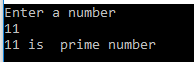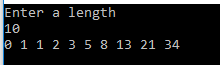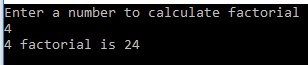# Func, Action And Local Function

Introduction

This article introduces you to how to use Func, Action and Local Function in C# 7 language. These methods are used to hide a method in another method or nested msethod. Func and Action are predefined generic delegates, which take zero to sixteen input parameters.

The basic difference between both is that Func always returns a value while Action doesn’t return a value.

Local function can be defined in the body of any method.

Both Func and Action delegates must be defined before they are called, while Local function can be defined later in the method.

Func

The Func<T,TResult> is a predefined generic delegate. It has zero to sixteen parameters and one out parameter. It always returns a value. As it is a function, first type parameters are the input arguments and the final type parameter is the return value. Func delegate type can be used with an anonymous method or lambda expression.

Here, we create an example, which checks whether a number is a prime number or not. This example uses Func<T,TResult> with a lambda expression to check prime number. The code snippet given below can be used for the same.

1. using static System.Console;
2. using System;
3.
5. {
6.     class Program
7.     {
8.         static void Main(string[] args)
9.         {
10.             Func<int, bool> checkPrimeNumber = x =>
11.             {
12.                 bool isPrime = false;
13.                 int i;
14.                 for (i = 2; i <= x - 1; i++)
15.                 {
16.                     if (x % i == 0)
17.                     {
18.                         isPrime = false;
19.                         break;
20.                     }
21.                 }
22.                 if (i == x)
23.                 {
24.                     isPrime = true;
25.                 }
26.                 return isPrime;
27.             };
28.
29.             WriteLine("Enter a number");
31.
33.             WriteLine(\$"{number } is {(isPrimeNumber ? "" : "not")} prime number");
35.         }
36.     }
37. }

As per the code snippet given above, Func<int,bool> has two parameters, the first parameter is an input parameter, while another is out parameter. It takes an int value as an input argument and returns a bool value.

Here, an input parameter named x goes to an anonymous function, which returns true, if the input is a prime number or not.

Now, run the Application. It shows the result as per the image given below.Figure 1: Output

Action

The Action is a predefined delegate, which has no return type. In other words, its return type is void. All the parameters of Action delegate are considered as the input parameters. It is more commonly used for things like List<T>.ForEach: execute the given action for each item in the list.

Here, we create an example, which prints a Fibonacci series. This example uses Action<T> with a lambda expression to print a Fibonacci series. The code snippet given below can be used for the same.

1. using System;
2. using static System.Console;
3.
4. namespace FibonacciSeriesApp
5. {
6.     class Program
7.     {
8.         static void Main(string[] args)
9.         {
10.             Action<int> GetFibonacciSeries = x =>
11.              {
12.                  int starter = 0, next = 1, number = 0;
13.                  Write(\$"{starter} {next}");
14.                  for (int i = 2; i < x; i++)
15.                  {
16.                      number = starter + next;
17.                      Write(\$" {number}");
18.                      starter = next;
19.                      next = number;
20.                  }
21.              };
22.             WriteLine("Enter a length");
24.             GetFibonacciSeries(num);
26.         }
27.     }
28. }

As per the code snippet given above, Action<int> has one parameter, which takes an input argument as int type.

Here, an input parameter named x goes to an anonymous function, which prints a Fibonacci series of x length.

Now, run the Application. It shows the result, as shown in the image given below.Figure 2: Output

Local Function

The Local function is a new concept introduced in C# 7. The Local function can be defined in the body of any method and property’s getter and setter. All the arguments and the local variables in the outer function are in scope of local function. These local functions can use ref and out parameters.

Here, we create an example, whichs calculates a factorial number of a given number. This example uses a local function named GetFactorial to calculate factorial of the number. The code snippet given below can be used for the same.

1. using System;
2. using static System.Console;
3.
4. namespace LocalFunctionApp
5. {
6.     class Program
7.     {
8.         static void Main(string[] args)
9.         {
10.             WriteLine("Enter a number to calculate factorial");
12.             long fact = GetFactorial(num);
13.             WriteLine(\$"{num} factorial is {fact}");
14.             long GetFactorial(int number)
15.             {
16.                 return number == 0 ? 1 : number * GetFactorial(number - 1);
17.             }
19.         }
20.     }
21. }

As per the code snippet given above, GetFactorial is a local function in the Main method, which has a parameter and returns a long type value.

Now, run the Application. It shows the result, as shown below.Figure 3: Output